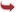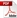International Journal of Scientific and Research Publications

#### IJSRP, Volume 6, Issue 9, September 2016 Edition [ISSN 2250-3153]Selection of Optimum Single Sampling Plans under Prior Binomial distribution by minimizing the Average acceptance Cost
M.Nirmala, R.Subramaniam and G.Uma
Abstract: This paper deals with the determination of Optimum Single Sampling Plans (n,c) based on the Bayesian Prior Binomial Distribution for various values of the Lot sizes ‘N’ by minimizing the average acceptance cost K(N, n, c, p) subject to the condition, the cost associated with a defective item which is accepted (A2) is very small, Producer’s Risk and Consumer’s Risk are minimized (or) power 1-β is maximized. The ideal Operating Characterizing Curve for a Bayesian Single Sampling Plan is also constructed by considering AQL and LQL for the optimum acceptance number ‘c’.
[VIEW FULL PAPER][DOWNLOAD]

Reference this Research Paper (copy & paste below code):

M.Nirmala, R.Subramaniam and G.Uma (2018); Selection of Optimum Single Sampling Plans under Prior Binomial distribution by minimizing the Average acceptance Cost; Int J Sci Res Publ 6(9) (ISSN: 2250-3153). http://www.ijsrp.org/research-paper-0916.php?rp=P575787